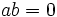# Primary ring

This article defines a property of commutative unital rings; a property that can be evaluated for a commutative unital ring
View all properties of commutative unital rings
VIEW RELATED: Commutative unital ring property implications | Commutative unital ring property non-implications |Commutative unital ring metaproperty satisfactions | Commutative unital ring metaproperty dissatisfactions | Commutative unital ring property satisfactions | Commutative unital ring property dissatisfactions
The property of being an ideal for which the quotient ring has this property is: primary ideal

## Definition

### Symbol-free definition

A commutative unital ring is termed a primary ring if it satisfies the following equivalent conditions:

• Whenever the product of two elements in it is zero, either the first element is zero, or the second element is nilpotent
• The zero ideal is a primary ideal
• The ring, as a module over itself, has a unique associated prime.

### Definition with symbols

A commutative unital ring$R$ is termed a primary ring is whenever$ab = 0$ in$R$, then either$a = 0$ or there exists a$n$ such that$b^n = 0$.